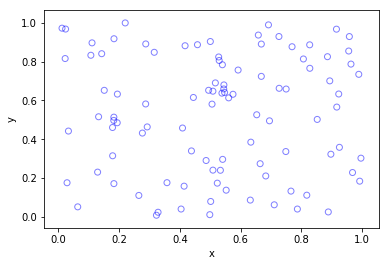## Simulating Poisson random variables – Survey of methods

In the previous post, I discussed how to sample or generate Poisson random variables or (more correctly) variates. I detailed a direct method that uses the fact that a Poisson stochastic process, which is directly related to a Poisson point process, has inter-arrival times that form independent and identically distributed exponential variables. This method in turn can be easily reformulated so it only uses (standard) uniform variables to generate Poisson random variables. It is an easy and popular sampling method, explaining why it is often used.

As elegant and precise as this simulation method is, it unfortunately decreases in speed as the Poisson parameter $$\lambda$$ increases. In a tutorial published in 1983, Brian D. Ripely, a major figure in spatial statistics, says this about the direct method:

This is simple, but has expected time proportional to $$\lambda$$. Some of its competitors use rejection methods with the envelope distribution that of the integer part of a continuous random variable, such as logistic, Laplace and normal mixed with exponential distributions.

We recall that acceptance-rejection or rejections methods involve simulating a random object, such as a random variable, by first simulating another random object of the same type that is easier to simulate, and then accepting or rejecting these random variables or variates based on a certain ratio. The distribution of the random object that is first simulated is called the envelope distribution. Such rejection methods are one way to simulate Poisson variables.

Consequently, the code of most computer functions for generating Poisson variables will have an if-statement, using the direct method for small parameter values and another method for large parameter values.

### Different methods

Over the years there have been different methods proposed for producing Poisson random variates. In the book Non-uniform random variate generation, Luc Devroye groups the different methods into five categories with his views, which I’ll briefly describe here:

1. Direct methods based on the homogeneous Poisson stochastic process having exponential inter-arrival times. These methods are simple, but the expected time is proportional to the Poisson parameter $$\lambda$$.
2. Inversion methods that search through a table of cumulative Poisson probabilities. Examples include the papers by Fishman (1976) and Atkinson (1979)*.
3. Methods that use the recursive properties of the Poisson distribution. The paper by Ahrens and Dieter (1974) uses this approach, and its expected time of completion is proportional to $$\log\lambda$$.
4. Acceptance-rejection (or rejection) methods that give relatively fast but simple algorithms. Such methods are proposed in the papers by Atkinson (1979)*, Ahrens and Dieter (1980) and Devroye (1981) or the technical report by Schmeiser and Kachitvichyanukul (1981).
5. Acceptance-complement methods that uses a normal distribution as the starting distribution, such as the paper by Ahrens and Dieter (1982). This method is fast, but the code is rather long.

*Atkinson had (at least) two papers on generating Poisson variates published in 1979, but I believe Devroye is referring to the first paper, because in the second paper Atkinson compares methods proposed by others.

For titles of the papers, see the Further reading section below.

## Methods implemented

In this section, I’ll state which proposed methods are used in various programming languages and numerical methods. I won’t go into the details how the methods work, as I’ll just cite the papers instead.

##### MATLAB

For small $$\lambda$$ values, the MATLAB function poissrnd uses the  direct method (based on inter-arrival times) with a while-loop.

For $$\lambda$$ values greater than fifteen, I believe that the MATLAB function poissrnd uses Algorithm PG from the 1974 paper by Ahrens and Dieter. But to come to this conclusion, I had to do some investigating. You can skip to the next section if you’re not interested, but now I’ll explain my reasoning.

The MATLAB documentation says it uses a method proposed by Ahrens and Dieter, but these two researchers developed a number of methods for generating Poisson variables. The MATLAB code cites Volume 2 of the classic series by Knuth, who says the method is due to Ahrens and Dieter, but he doesn’t give an exact citation in that section of the book. Confusingly, Knuth cites in his book a couple papers by Ahrens and Dieter for generating different random variates. (Knuth later cites a seemingly relevant 1980 paper by Ahrens and Dieter, but that details another method.)

Both the MATLAB code and Knuth cite the book by Devroye. In his book (Exercise 3.5.2), Devroye discusses one method, among others, from a 1974 paper by Ahrens and Dieter. Another hint is given by examining the code of the MATLAB function poissrnd, which reveals that it uses the function randg to generate gamma variables. In the Ahrens and Dieter 1974 paper, their Algorithm PG (for producing Poisson variates) uses gamma random variables, and it’s suggested to use a parameter value of $$7/8$$, as used by MATLAB and mentioned by Knuth, confirming this is the right paper.

In summary, for large $$\lambda$$ the function MATLAB uses Algorithm PG from the 1974 paper by Ahrens and Dieter, whereas it uses the direct method for small values.

##### R

In R, the function rpois use an algorithm outlined in the 1982 paper by Ahrens and Dieter. You can view the R source code here. The two cases for $$\lambda$$ (or $$\mu$$ in the paper) depend on whether $$\lambda$$ is greater than ten or not. For small $$\lambda$$, the R function rpois does not use the method based on inter-arrival times, but rather an inversion method based on a table of (cumulative) probabilities given by the Poisson probability distribution.

##### Python (NumPy)

In NumPy, the function numpy.random.poisson generates Poisson variates. The source code for the NumPy library is here, but for Poisson function the underlying code is actually written in C; see the distributions.c file located here. For small Poisson parameter $$\lambda$$, the code uses the direct method; see the function random_poisson_mult in the code.

For Poisson parameter $$\lambda \geq 10$$, the comments in the code reveal that it uses a method from a 1993 paper by Hörmann; see Algorithm PTRS on page 43 of the paper. This is a transformation method, which for NumPy is implemented in the C code as the function random_poisson_ptrs. The method, which Hörmann calls the transformed rejection with squeeze, combines inversion and rejection methods.

##### Octave

Octave is intended to be a GNU clone of MATLAB, so you would suspect it uses the same methods as MATLAB for generating Poisson random variates. But the Octave function poissrnd uses different methods. The code reveals it generates the Poisson variates with a function called prand. It considers different cases depending on the value of the Poisson parameter $$\lambda$$ as well as whether a single variable (that is, a scalar) or vector or matrix of Poisson variates are being generated.

In total, the Octave function prand uses five different methods. For two of the methods, the documentation cites methods from the classic book Numerical Recipes in C (the 1992 edition); see next section. To generate a single Poisson variate with Poisson parameter $$\lambda \leq 12$$, the Octave function prand uses the direct method based on inter-arrival times.

##### Numerical Recipes (Fortran, C and C++)

The Numerical Recipes is a classic book by Press, Teukolsky, Vetterling and Flannery on numerical methods. The books comes in different editions reflecting different publication years and computer languages. (In the first two editions of the book, the authors implemented the algorithms respectively in Fortran and C.)

For generating Poisson variates, the book contents seems to have not changed over the editions that I looked at, which covered the programming languages Fortran (77 and 90), C, and C++. The authors cover Poisson generation in Section 7.3 in the Fortran and C editions. In the third edition of Numerical Recipes, they implement their methods in C++ in Section 7.3.12.

For small values of Poisson parameter $$\lambda$$, Numerical Recipes uses the direct method. For $$\lambda >12$$ values, an acceptance-rejection method is used, which relies upon finding a continuous version of the discrete Poisson probability distribution.

##### GSL Library (C)

In the GSL library, one can use the function gsl_ran_poisson, which uses the the direct method of exponential times. The code, which can be viewed here, cites simply Knuth (presumably the second volume).

##### NAG Library (C)

Although I didn’t see the code, it appears that the function nag_rand_poisson (g05tjc ) in the NAG library also uses the direct method, based on the material in the second volume of series by Knuth. But in a 1979 paper Atkinson says that the NAG library uses a method from the 1974 paper by Ahrens and Dieter.

##### MKL library (C)

In the MKL C library written by Intel, they seem to have three functions or methods for generating Poisson variates.

The first function is called VSL_RNG_METHOD_POISSON_PTPE, which does the following for a Poisson distribution with parameter $$\Lambda$$:

If Λ ≥ 27, random numbers are generated by PTPE method. Otherwise, a combination of inverse transformation and table lookup methods is used. The PTPE method is a variation of the acceptance/rejection method that uses linear (on the fraction close to the distribution mode) and exponential (at the distribution tails) functions as majorizing functions. To avoid time-consuming acceptance/rejection checks, areas with zero probability of rejection are introduced and a squeezing technique is applied.

This function uses the so-called PTPE method, which is outlined in a 1981 technical report by Schmeiser and Kachitvichyanukul.

The second function is called VSL_RNG_METHOD_POISSON_POISNORM, which does the following :

If Λ < 1, the random numbers are generated by combination of inverse transformation and table lookup methods. Otherwise, they are produced through transformation of the normally distributed random numbers.

The third function is called VSL_RNG_METHOD_POISSONV_POISNORM, which does the following:

If Λ < 0.0625, the random numbers are generated by inverse transformation method. Otherwise, they are produced through transformation of normally distributed random numbers.

## cuRAND (C)

Finally, Nvidia’s CUDA Random Number Generation library (cuRAND) has a function for generating Poisson variates. To see the C code, copies of it can be found in various GitHub repositories, such as this one. The cuRAND function curand_poisson uses the direct function for Poisson parameter values  less than 64. For parameters values greater than 4000, it uses a normal approximation (rounded to the nearest integer).

For other values, the function curand_poisson uses an a rejection method based on an approximation of the incomplete gamma function; see the function curand_poisson_gammainc. The book by Fishman is cited; see Section 8.16.

For various Poisson simulation methods, see the stochastic simulation books by Devroye (Section X.3) or Fishman (Section 8.16). The book by Gentle (Section 5.2.8) also briefly covers Poisson variables. Kuhl wrote a paper on the history of generating random variates. It has some interesting historical points, but I was not entirely impressed by the quality or detail of the paper.

Of course, it’s a good idea to look at the citations that the different functions use.

Finally, here is a list of the papers I mentioned in this post:

• 1974, Ahrens and Dieter, Computer methods for sampling from gamma, beta, poisson and bionomial distributions;
• 1976, Fishman, Sampling from the Poisson distribution on a computer;
• 1979, Atkinson, The computer generation of Poisson random variables;
• 1979, Atkinson, Recent developments in the computer generation of Poisson random variables;
• 1980, Ahrens and Dieter, Sampling from binomial and Poisson distributions: a method with bounded computation times;
• 1980, Devroye, The Computer Generation of Poisson Random Variables;
• 1981, Schmeiser and Kachitvichyanukul, Poisson Random Variate Generation;
• 1982, Ahrens and Dieter, Computer generation of Poisson deviates from modified normal distributions;
• 1983, Ripley, Computer Generation of Random Variables: A Tutorial;
• 1993, Hörmann, The transformed rejection method for generating Poisson random variable.

## Simulating a Cox point process based on a Poisson line process

In the previous post, I described how to simulate a Poisson line process, which in turn was done by using insight from an earlier post on the Bertrand paradox.

Now, given a Poisson line process, for each line, if we generate an independent one-dimensional Poisson point point process on each line, then we obtain an example of a Cox point process. Cox point processes are also known as doubly stochastic Poisson point processes. On the topic of names, Guttorp and Thorarinsdottir argue that it should be called the Quenouille point process, as Maurice Quenouille introduced an example of it before Sir David Cox, but I opt for the more common name.

## Cox point proceesses

A Cox point process is a generalization of a Poisson point process. It is created by first considering a non-negative random measure, sometimes called a driving measure. Then a Poisson point process, which is independent of the random driving measure, is generated by using the random measure as its intensity or mean measure.

The driving measure of a Cox point process can be, for example, a non-negative random variable or field multiplied by a Lebesgue measure. In our case, the random measure is the underlying Poisson line process coupled with the Lebesgue measure on the line (that is, length).

Cox processes form a very large and general family of point processes, which exhibit clustering. In previous posts, I have covered two special cases of Cox point processes: the Matérn and Thomas cluster point processes. These are, more specifically, examples of a Neyman-Scott point process, which is a special case of a shot noise Cox point process. These two point processes are fairly easy to simulate, but that’s not the case for Cox point processes in general. Some are considerably easier than others.

## Motivation

I will focus on simulating the Cox point process formed from a Poisson line process with homogeneous Poisson point processes. I do this for two reasons. First, it’s easy to simulate, given we can simulate a Poisson line process. Second, it has been used and studied recently in the mathematics and engineering literature for investigating wireless communication networks in cities, where the streets correspond to Poisson lines; for example, see these two preprints:

Incidentally, I don’t know what to call this particular Cox point process. A Cox line-point process? A Cox-Poisson line-point process? But it doesn’t matter for simulation purposes.

## Method

We will simulate the Cox (-Poisson line-) point process on a disk. Why a disk? I suggest reading the previous posts on the Poisson line process the Bertrand paradox for why the disk is a natural simulation window for line processes.

Provided we can simulate a Poisson line process, the simulation method is quite straightforward, as I have essentially already described it.

##### Line process

First simulate a Poisson line process on a disk. We recall that for each line of the line process, we need to generate two independent random variables $$\Theta$$ and $$P$$ describing the position of the line. The first random variable $$\Theta$$ gives the line orientation, and it is a uniform random variable on the interval $$(0,2\pi)$$.

The second random variables $$P$$ gives the distance from the origin to the disk edge, and it is a uniform random variable on the interval $$(0,r)$$, where $$r$$ is the radius of the disk. The distance from the point $$(\Theta, P)$$ to the disk edge (that is, the circle) along the chord is:

$$Q=\sqrt{r^2-P^2}.$$

The endpoints of the chord (that is, the points on the disk edge) are then:

Point 1: $$X_1=P \cos \Theta+ Q\sin \Theta$$, $$Y_1= P \sin \Theta- Q\cos \Theta$$,

Point 2: $$X_2=P \cos \Theta- Q\sin \Theta$$, $$Y_2= P \sin \Theta+Q \cos \Theta$$.

The length of the line segment is $$2 Q$$. We can say this random line is described by the point $$(\Theta,P)$$.

##### One-dimensional Poisson point process

For each line (segment) in the line process, simulate a one-dimensional Poisson point process on it. Although I have never discussed how to simulate a one-dimensional (homogeneous) Poisson point process, it’s essentially one dimension less than simulating a homogeneous Poisson point process on a rectangle.

More specifically, given a line segment $$(\Theta,P)=(\theta,p)$$, you simulate a homogeneous Poisson point process with intensity $$\mu$$ on a line segment with length $$2 q$$, where $$q=\sqrt{r^2-p^2}$$. (I am now using lowercase letters to stress that the line is no longer random.) To simulate the homogeneous Poisson point process, you generate a Poisson random variable with parameter $$2 \mu q$$.

Now you need to place the points uniformly on the line segment. To do this, consider a single point on a single line. For this point, generate a single uniform variable $$U$$ on the interval $$(-1,1)$$. The tricky part is now getting the Cartesian coordinates right. But the above expressions for the endpoints suggest that the single random point has the Cartesian coordinates:

$$x=p \cos \theta+ U q\sin \theta$$, $$y=p \sin \theta- U q\cos \theta$$.

The two extreme cases of the uniform random variable $$U$$ (that is, $$U=-1$$ and $$U=1$$) correspond to the two endpoints of the line segment. We recall that $$Q$$ is the distance from the midpoint of the line segment to the disk edge along the line segment, so it makes sense that we want to vary this distance uniformly in order to uniformly place a point on the line segment. This uniform placement step is done for all the points of the homogeneous Point process on that line segment.

You repeat this procedure for every line segment. And that’s it: a Cox point process built upon a Poisson line process.

## Results

##### MATLAB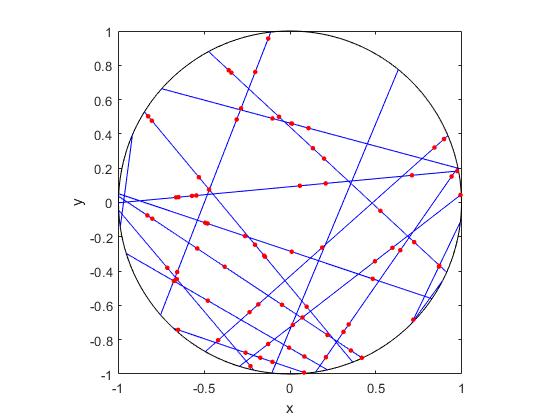##### R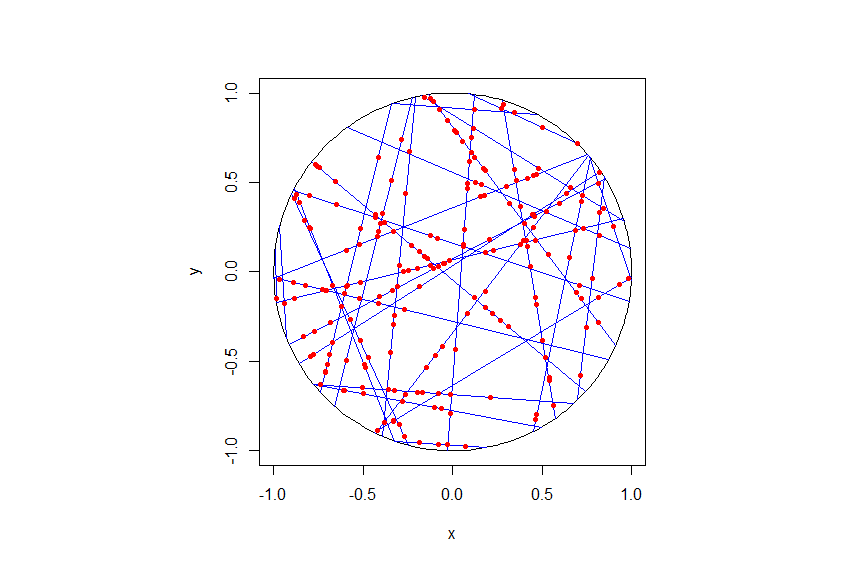##### Python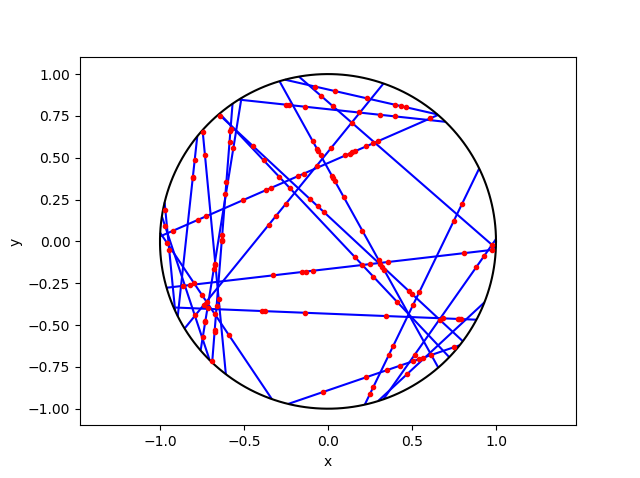## Code

As always, the code from all my posts is online. For this post, I have written the code in MATLAB, R and Python.

For the first step, the reading material is basically the same as that for the Poisson line process, which overlaps with that of the Bertrand paradox. For the one-dimensional Poisson point process, we can use the reading material on the homogeneous Poisson point process on a rectangle.

For general Cox point processes, I recommend starting with the following: Chapter 6 in the monograph Poisson processes by Kingman; Chapter 5 in Statistical Inference and Simulation for Spatial Point Processes by Møller and Waagepetersen; and Section 5.2 in Stochastic Geometry and its Applications by Chiu, Stoyan, Kendall and Mecke. For a much more mathematical treatment, see Chapter 13 in Lectures on the Poisson Process by Last and Penrose.

For this particularly Cox point process, see the two aforementioned preprints, located here and here.

## Simulating a Poisson line process

Instead of points, we can consider other objects randomly scattered on some underlying mathematical space. If we take a Poisson point process, then we can use (infinitely long) straight lines instead of points, giving a Poisson line process. Researchers have studied and used this random object to model physical phenomena. In this post I’ll cover how to simulate a homogeneous Poisson line process in MATLAB, R and Python. The code which can be downloaded from here

## Overview

For simulating a Poisson line process, the key question is how to randomly position the lines.  This is related to a classic problem in probability theory called the Bertrand paradox.  I discussed this illustration in probability in a previous post, where I also included code for simulating it. I highly recommend reading that post first.

The Bertrand paradox involves three methods for positioning random lines. We use Method 2 to achieve a uniform positioning of lines, meaning the number of lines and orientation of lines is statistically uniform. Then it also makes sense to use this method for simulating a homogeneous (or uniform) Poisson line process.

We can interpret a line process as a point process. For a line process on the plane $$\textbf{R}^2$$, it can be described by a point process on $$(0,\infty)\times (0,2\pi)$$, which is an an infinitely long cylinder. In other words, the Poisson line process can be described as a Poisson point process.

For simulating a Poisson line process, it turns out the disk is the most natural setting. (Again, this goes back to the Bertrand paradox.) More specifically, how the (infinitely long) lines intersect a disk of a fixed radius $$r>0$$. The problem of simulating a Poisson line process reduces to randomly placing chords in a disk. For other simulation windows in the plane, we can always bound any non-circular region with a sufficiently large disk.

## Steps

##### Number of lines

Of course, with all things Poisson, the number of lines will be  a Poisson random variable, but what will its parameter be? This homogeneous (or uniform) Poisson line process forms a one-dimensional homogeneous (or uniform) Poisson point process around the edge of the disk with a circumference $$2 \pi r$$. Then the number of lines is simply a Poisson variable with parameter $$\lambda 2 \pi r$$.

##### Locations of points

To position a single line uniformly in a disk, we need to generate two uniform random variables. One random variable gives the angle describing orientation of the line, so it’s a uniform random variable $$\Theta$$ on the interval $$(0,2\pi)$$.

The other random variable gives the distance from the origin to the disk edge, meaning it’s a uniform random variable $$P$$ on the interval $$(0,r)$$, where $$r$$ is the radius of the disk.  The random radius and its perpendicular chord create a right-angle triangle.  The distance from the point $$(\Theta, P)$$ to the disk edge (that is, the circle) along the chord is:

$$Q=\sqrt{r^2-P^2}.$$

The endpoints of the chord (that is, the points on the disk edge) are then:

Point 1: $$X_1=P \cos \Theta+ Q\sin \Theta$$, $$Y_1= P \sin \Theta- Q\cos \Theta$$,

Point 2: $$X_2=P \cos \Theta- Q\sin \Theta$$, $$Y_2= P \sin \Theta+Q \cos \Theta$$.

## Code

I have implemented the simulation procedure in MATLAB, R and Python, which, as usual, are all very similar. I haven’t put my code here, because the software behind my website keeps mangling it.  As always, I have uploaded my code to a repository; for this post, it’s in this directory.

I have written the code in R, but I wouldn’t use it in general. That’s because if you’re using R, then, as I have said before, I strongly recommend using the powerful spatial statistics library spatstat. For a simulating Poisson line process, there’s the function rpoisline.

The chief author of spatstat, Adrian Baddeley, has written various lectures and books on the related topics of point processes, spatial statistics, and geometric probability. In this post, he answered why the angular coordinates have to be chosen uniformly.

### Results

MATLAB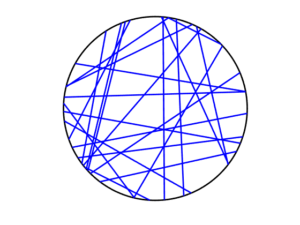R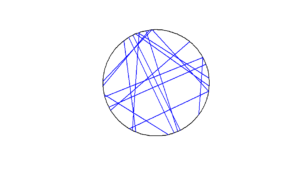Python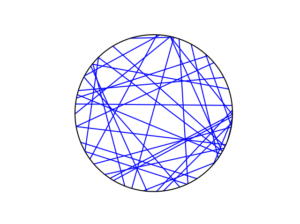To read about the Poisson line process, it’s best to start with the Bertrand problem, which is covered in many works on geometric probability and related fields. A good and recent introduction is given by Calka in (Section 1.3) of the lectures titled Stochastic Geometry: Modern Research Frontiers, which were edited by Coupier and published by Springer.  Also see, for example, problem 1.2 in Geometrical Probability by Kendall and Moran or page 44 in Geometric Probability by Solomon.

For the Poisson line process, I highly recommend Section 7.2 in the monograph Poisson processes by Kingman. Also see Example 8.2 in the standard textbook Stochastic Geometry and its Applications by Chiu, Stoyan, Kendall and Mecke. The Poisson line process book also appears in Exercise 5.2 in the book Stochastic Simulation – Algorithms and Analysis by  Asmussen and Glynn.

For online resources, this set of lectures by Christian Lantuéjoul
covers the Poisson line process. Wilfrid Kendall covers the Poisson line process in this talk in relation to so-called Poisson cities.

Mathematical paradoxes are results or observations in mathematics that are (seemingly) conflicting, unintuitive, incomprehensible, or just plain bizarre. They come in different flavours, such as those that play with notions of infinity, which means they often make little or no sense in a physical world. Other paradoxes, particularly those in probability, serve as a lesson that the problem needs to be posed in a precise manner. The Bertrand paradox is one of these.

Joseph Bertrand posed the original problem in his 1889 book Calcul des probabilités, which is available online (albeit in French). It’s a great illustrative problem involving simple probability and geometry, so it often appears in literature on the (closely related) mathematical fields of geometric probability and integral geometry.

Based on constructing a random chord in a circle, the paradox involves a single mathematical problem with three reasonable but different solutions. It’s less a paradox and more a cautionary tale. It’s really asking the question: What do you mean by random?

Consequently, over the years the Bertrand paradox has inspired debate, with papers arguing what the true solution is. I recently discovered it has even inspired some passionate remarks on the internet; read the comments at www.bertrands-paradox.com.

But I am less interested in the different interpretations or philosophies of the problem. Rather, I want to simulate the three solutions. This is not very difficult, provided some trigonometry and knowledge from a previous post, where I describe how to simulate a (homogeneous) Poisson point process on the disk.

I won’t try to give a thorough analysis of the solutions, as there are much better websites doing that. For example, this MIT website gives a colourful explanation with pizza and fire-breathing monsters. The Wikipedia article also gives a detailed though less creative explanation for the three solutions.

My final code in MATLAB, R and Python code is located here.

### The Problem

Bertrand considered a circle with an equilateral triangle inscribed it. If a chord in the circle is randomly chosen, what is the probability that the chord is longer than a side of the equilateral triangle?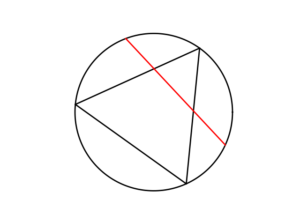### The Solution(s)

Bertrand argued that there are three natural but different methods to randomly choose a chord, giving three distinct answers. (Of course, there are other methods, but these are arguably not the natural ones that first come to mind.)

##### Method 1: Random endpoints

On the circumference of the circle two points are randomly (that is, uniformly and independently) chosen, which are then used as the two endpoints of the chord.

The probability of this random chord being longer than a side of the triangle is one third.

A radius of the circle is randomly chosen (so the angle is chosen uniformly), then a point is randomly (also uniformly) chosen along the radius, and then a chord is constructed at this point so it is perpendicular to the radius.

The probability of this random chord being longer than a side of the triangle is one half.

##### Method 3: Random midpoint

A point is randomly (so uniformly) chosen in the circle, which is used as the midpoint of the chord, and the chord is randomly (also uniformly) rotated.

The probability of this random chord being longer than a side of the triangle is one quarter.

### Simulation

All three answers involve randomly and independently sampling two random variables, and then doing some simple trigonometry. The setting naturally inspires the use of polar coordinates. I assume the circle has a radius $$r$$ and a centre at the point origin $$o$$. I’ll number the end points one and two.

In all three solutions we need to generate uniform random variables on the interval $$(0, 2\pi)$$ to simulate random angles. I have already done this a couple of times in previous posts such as this one.

##### Method 1: Random endpoints

This is probably the most straightforward solution to simulate. We just need to simulate two uniform random variables $$\Theta_1$$ and $$\Theta_2$$ on the interval $$(0, 2\pi)$$ to describe the angles of the two points.

The end points of the chord (in Cartesian coordinates) are then simply:

Point 1: $$X_1=r \cos \Theta_1$$, $$Y_1=r \sin \Theta_1$$,

Point 2: $$X_2=r \cos \Theta_2$$, $$Y_2=r \sin \Theta_2$$.

This method also involves generating two uniform random variables. One random variable $$\Theta$$ is for the angle, while the other $$P$$ is the random radius, which means generating the random variable $$P$$ on the interval $$(0, r)$$.

I won’t go into the trigonometry, but the random radius and its perpendicular chord create a right-angle triangle. The distance from the point $$(\Theta, P)$$ to the circle along the chord is:

$$Q=\sqrt{r^2-P^2}.$$

The endpoints of the chord are then:

Point 1: $$X_1=P \cos \Theta+ Q\sin \Theta$$, $$Y_1= P \sin \Theta- Q\cos \Theta$$,

Point 2: $$X_2=P \cos \Theta- Q\sin \Theta$$, $$Y_2= P \sin \Theta+Q \cos \Theta$$.

Take note of the signs in these expressions.

##### Method 3: Random midpoint

This method requires placing a point uniformly on a disk, which is also done when simulating a homogeneous Poisson point process on a disk, and requires two random variables $$\Theta’$$ and $$P’$$. Again, the angular random variable $$\Theta’$$ is uniform.

The other random variable $$P’$$ is not uniform. For $$P’$$, we generate a random uniform variable on the unit interval $$(0,1)$$, and then we take the square root of it. We then multiply it by the radius, generating a random variable between $$0$$ and $$r$$. (We must take the square root because the area element of a sector is proportional to the radius squared, and not the radius.) The distribution of this random variable is an example of the triangular distribution.

The same trigonometry from Method 2 applies here, which gives the endpoints of the chord as:

Point 1: $$X_1=P’ \cos \Theta’+ Q’\sin \Theta’$$, $$Y_1= P’ \sin \Theta’- Q’\cos \Theta’$$,

Point 2: $$X_2=P’\cos \Theta’- Q’\sin \Theta’$$, $$Y_2= P’\sin \Theta’+Q’ \cos \Theta’$$,

where $$Q’:=\sqrt{r^2-{P’}^2}$$. Again, take note of the signs in these expressions.

### Results

To illustrate how the three solutions are different, I’ve plotted a hundred random line segments and their midpoints side by side. Similar plots are in the Wikipedia article.

#####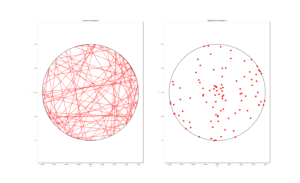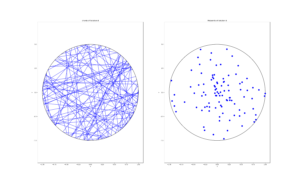##### Method 3: Random midpoint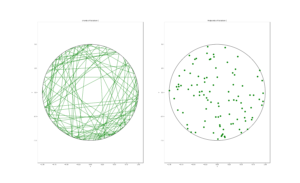### Conclusion

For the chord midpoints, we know and can see that Method 3 gives uniform points, while Method 2 has a concentration of midpoints around the circle centre. Method 1 gives results that seem to somewhere between Method 2 and 3 in terms of clustering around the circle centre.

For the chords, we see that Method 3 results in fewer chords passing through the circle centre. Methods 1 and 2 seem to give a similar number of lines passing through this central region.

It’s perhaps hard to see, but it can be shown that Method 2 gives the most uniform results. By this, I mean that the number of lines and their orientations statistically do not vary in different regions of the circle.

We can now position random lines in uniform manner. All we need now is a Poisson number of lines to generate something known as a Poisson line process, which will be the focus of the next post.

I’ve already mentioned that there are some good websites on the topic of the Bertrand paradox. For example:

web.mit.edu/tee/www/bertrand

www.cut-the-knot.org/bertrand.shtml

mathworld.wolfram.com/BertrandsProblem.html

Various authors have mentioned or discussed the Bertrand paradox in books on the related subjects of geometric probability, integral geometry and stochastic geometry. A good and recent introduction is given by Calka in Section 1.3 of the published lectures Stochastic Geometry: Modern Research Frontiers.

Other classic books that cover the topic including, for example, see Problem 1.2 in Geometrical Probability by Kendall and Moran. (Despite Maurice G. Kendall writing a book on geometric probability, he was not related to stochastic geometry pioneer David G Kendall.) It’s also discussed on page 44 in Geometric Probability by Solomon. For a book that involves more advance knowledge of geometry and (abstract) algebra, see Chapter 3 in Integral Geometry and Geometric Probability by Santaló.

The Bertrand paradox is also in The Pleasures of Probability by Isaac. It’s covered in a non-mathematical way in the book Paradoxes from A to Z by Clark. Edwin Jaynes studied the problem and proposed a solution in a somewhat famous 1973 paper, titled The Well-Posed Problem.

The original problem can be read in French in Bertrand’s work, which is available online here or here (starting at the bottom of page 4).

### Code

The MATLAB, R and Python code can be found here. In the code, I have labelled the methods A, B and C instead 1, 2 and 3.

## Thinning point processes

One way to create new point processes is to apply thinning to a point process. As I mentioned in a previous post on point process operations, thinning is a random operation applied to the points of an underlying point process, where the points are thinned (or removed) or retained (or kept) according to some probabilistic rule. Both the thinned and retained points form two separate point processes, but one usually focuses on the retained points. Given an underlying point process, the complexity of the thinning rule will result in different types of point processes.

## Thinning types

Thinning can be statistically independent or dependent, meaning that the probability of thinning any point is either independent or dependent of thinning other points. The more tractable case is statistically independent thinning, which is the thinning type covered here. We can further group this thinning into two types based on whether the thinning rule depends on the locations of the point. (I use the word location, instead of point, to refer to where a point of a point process is located on the underlying mathematical space on which the point process is defined.)

##### Spatially independent thinning

The simplest thinning operation is one that does not depend on point locations. This thinning is sometimes referred to as $$p$$-thinning, where the constant $$p$$ has the condition $$0\leq p \leq 1$$ because it is the probability of thinning a single point. Simply put, the probability of a point being thinned does not depend on the point locations.

Example

We can liken the thinning action to flipping a biased coin with probability of $$p$$ for heads (or tails) for each point of the underlying point process, and then removing the point if a head (or tails) occurs. If there were a constant number $$n$$ of points of the underlying point process, then the number of thinned (or retained) points will form a binomial random variable with parameters $$n$$ and $$p$$ (or $$1-p$$).

Simulation

Simulating this thinning operation is rather straightforward. Given a realization of a point process, for each point a uniform random variable on the interval $$(0,1)$$, simply generate or simulate, and if this random variable is less than $$p$$, remove the point. In the code section below, I have shown how this thinning operation is implemented.

##### Spatially dependent thinning

To generalize the idea of $$p$$-thinning, we can simply require that the thinning probability of any point depends on its location $$x$$, which gives us the concept of $$p(x)$$-thinning. (I write a single $$x$$ to denote a point on the plane, that is $$x\in \mathbb{R}^2$$, instead of writing, for example, the $$x$$ and $$y$$ and coordinates separately.) More precisely, the probability of thinning a point is given by a function $$p(x)$$ such that $$0 \leq p(x)\leq 1$$, but all point thinnings occur independently of each other. In other words, this is a spatially dependent thinning that is statistically independent.

Example

I’ll illustrate the concept of spatially dependent thinning with a somewhat contrived example. We assume that the living locations of all the people in the world form a point process on a (slightly squashed) sphere. Let’s say that Earth has become overpopulated, particularly in the Northern Hemisphere, so we decide to randomly choose people and send them off to another galaxy, but we do it based on how far they live from the North Pole. The thinning rule is, for example, $$p(x)= \exp(- |x|^2/s^2)$$, where $$|x|$$ is the distance to the North Pole and $$s>0$$ is some constant for distance scaling.

Put another way, a person at location $$x$$ flips a biased coin with the probability of, say, heads being equal to $$p(x)=\exp(- |x|^2/s^2)$$. If a head comes up, then that person is removed from the planet. With the maximum of $$p(x)$$ is at the North Pole, we can see that the lucky (or unlucky?) people in countries like Australia, NZ, South Africa, Argentina and Chile, are more likely not to be sent off (that is, thinned). For people who live comparable distances from the North Pole, the removal probabilities are similar in value, yet the events of being remove remain independent. For example, the probabilities of removing any two people from Lesotho are similar in value, but these two random events are still completely independent of each other.

Simulation

Simulating a spatially dependent thinning is just slightly more involved than the spatially independent case. Given a realization of a point process, for each point at, say, $$x$$, simply generate or simulate a uniform random variable on the interval $$(0,1)$$, and if this random variable is less than $$p(x)$$, remove the point.

In the code section, I have shown how this thinning operation is implemented with an example like the above one, but on a rectangular region of Cartesian space. In this setting, the maximum of $$p(x)$$ is at the origin, resulting in more points being thinned in this region.

## Thinning a Poisson point process

Perhaps not surprisingly, under the thinning operation the Poisson point process exhibits a closure property, meaning that a Poisson point process thinned in a certain way gives another Poisson point process.  More precisely, if the thinning operation is statistically independent, then the resulting point process formed from the retained points is also a Poisson point process, regardless if it is spatially independent or dependent thinning. The resulting intensity (interpreted as the average density of points) of this new Poisson point process has a simple expression.

##### Homogeneous case

For a spatially independent $$p$$-thinning, if the original (or underlying) Poisson point process is homogeneous with intensity $$\lambda$$, then the point process formed from the retained points is a homogeneous Poisson point process with intensity $$\lambda$$.  (There are different ways to prove this, but you can gain some intuition behind the proof by conditioning on the Poisson number of points and then applying the total law of probability. Using generating functions helps.)

##### Inhomogeneous case

More generally, if we apply a spatially dependent $$p(x)$$-thinning to a  Poisson point process has a intensity $$\lambda$$, then the retained points form a  an inhomogeneous or nonhomogeneous Poisson point process with $$\lambda p(x)$$,  due to the spatial dependence in the thinning function $$p(x)$$. This gives a way to simulate such Poisson point processes, which I’ll cover in another post.

##### Splitting

We can see by symmetry that if we look at the thinned points, then the resulting point process is also a Poisson point process, but with intensity $$(1-p(x))\lambda$$. The retained and thinned points both form Poisson point processes, but what is really interesting is these two point processes are independent of each other.  This means that any random configuration that occurs among the retained points is independent of any configurations among the thinned points.

This ability to split a Poisson point processes into independent ones is sometimes called the splitting property.

## Possible applications

Thinning point processes has the immediate application of creating new point processes. It can also be used to randomly generate two point processes from one. In network applications, a simple example is using the thinning procedure to model random sleep schemes in wireless networks, where random subsets of the network have been powered down.

## Code

All code from my posts, as always, can be found on the my GitHub repository. The code for this post is located here.

##### Spatially independent thinning

I have implemented in code the simple $$p$$-thinning operation applied to a Poisson point process on a rectangle, but in theory any point process can be used for the underlying point process that is thinned.

MATLAB

%Simulation window parameters
xMin=-1;xMax=1;
yMin=-1;yMax=1;
xDelta=xMax-xMin;yDelta=yMax-yMin; %rectangle dimensions
areaTotal=xDelta*yDelta; %area of rectangle

%Point process parameters
lambda=100; %intensity (ie mean density) of the Poisson process

%Thinning probability parameters
sigma=1;
p=0.25; %thinning probability

%Simulate Poisson point process
numbPoints=poissrnd(areaTotal*lambda);%Poisson number of points
xx=xDelta*(rand(numbPoints,1))+xMin;%x coordinates of Poisson points
yy=xDelta*(rand(numbPoints,1))+yMin;%y coordinates of Poisson points

%Generate Bernoulli variables (ie coin flips) for thinning
booleThinned=rand(numbPoints,1)>p; %points to be thinned
booleRetained=~booleThinned; %points to be retained

%x/y locations of thinned points
xxThinned=xx(booleThinned); yyThinned=yy(booleThinned);
%x/y locations of retained points
xxRetained=xx(booleRetained); yyRetained=yy(booleRetained);

%Plotting
plot(xxRetained,yyRetained,'bo'); %plot retained points
hold on; plot(xxThinned,yyThinned,'ro'); %plot thinned points
xlabel('x');ylabel('y');


R

#Simulation window parameters
xMin=-1;xMax=1;
yMin=-1;yMax=1;
xDelta=xMax-xMin;yDelta=yMax-yMin; #rectangle dimensions
areaTotal=xDelta*yDelta;

#Point process parameters
lambda=100; #intensity (ie mean density) of the Poisson process

#Thinning probability
p=0.25;

#Simulate a Poisson point process
numbPoints=rpois(1,areaTotal*lambda);#Poisson number of points
xx=xDelta*runif(numbPoints)+xMin;#x coordinates of Poisson points
yy=xDelta*runif(numbPoints)+yMin;#y coordinates of Poisson points

#Generate Bernoulli variables (ie coin flips) for thinning
booleThinned=runif(numbPoints)>p; #points to be thinned
booleRetained=!booleThinned; #points to be retained

#x/y locations of thinned points
xxThinned=xx[booleThinned]; yyThinned=yy[booleThinned];
#x/y locations of retained points
xxRetained=xx[booleRetained]; yyRetained=yy[booleRetained];

#Plotting
par(pty="s")
plot(xxRetained,yyRetained,'p',xlab='x',ylab='y',col='blue'); #plot retained points
points(xxThinned,yyThinned,col='red'); #plot thinned points



Of course, as I have mentioned before, simulating a spatial point processes in R is even easier with the powerful spatial statistics library spatstat.  With this library, thinning can be done in quite a general way by using the function rthin.

Python

import numpy as np; #NumPy package for arrays, random number generation, etc
import matplotlib.pyplot as plt

#Simulation window parameters
xMin=-1;xMax=1;
yMin=-1;yMax=1;
xDelta=xMax-xMin;yDelta=yMax-yMin; #rectangle dimensions
areaTotal=xDelta*yDelta;

#Point process parameters
lambda0=100; #intensity (ie mean density) of the Poisson process

#Thinning probability
p=0.25;

#Simulate a Poisson point process
numbPoints = np.random.poisson(lambda0*areaTotal);#Poisson number of points
xx = np.random.uniform(0,xDelta,((numbPoints,1)))+xMin;#x coordinates of Poisson points
yy = np.random.uniform(0,yDelta,((numbPoints,1)))+yMin;#y coordinates of Poisson points

#Generate Bernoulli variables (ie coin flips) for thinning
booleThinned=np.random.uniform(0,1,((numbPoints,1)))>p; #points to be thinned
booleRetained=~booleThinned; #points to be retained

#x/y locations of thinned points
xxThinned=xx[booleThinned]; yyThinned=yy[booleThinned];
#x/y locations of retained points
xxRetained=xx[booleRetained]; yyRetained=yy[booleRetained];

#Plotting
plt.scatter(xxRetained,yyRetained, edgecolor='b', facecolor='none', alpha=0.5 );
plt.scatter(xxThinned,yyThinned, edgecolor='r', facecolor='none', alpha=0.5 );
plt.xlabel("x"); plt.ylabel("y");
plt.show();

##### Spatially dependent thinning

I have implemented in code a $$p(x)$$-thinning operation with the function $$p(x)=\exp(-|x|^2/s^2)$$, where $$|x|$$ is the Euclidean distance from $$x$$ to the origin. This small changes means that in the code there will be a vector or array of $$p$$ values instead of a single $$p$$ value in the section where the uniform random variables are generated and compared said $$p$$ values.  (Lines 24, 26 and 28 respectively in the MATLAB, R and Python code presented below.)

Again, I have applied thinning to a Poisson point process on a rectangle, but in theory any point process can be used for the underlying point process.

MATLAB

%Simulation window parameters
xMin=-1;xMax=1;
yMin=-1;yMax=1;
xDelta=xMax-xMin;yDelta=yMax-yMin; %rectangle dimensions
areaTotal=xDelta*yDelta; %area of rectangle

%Point process parameters
lambda=100; %intensity (ie mean density) of the Poisson process

%Thinning probability parameters
sigma=0.5; %scale parameter for thinning probability function
%define thinning probability function
fun_p=@(s,x,y)(exp(-(x.^2+y.^2)/s^2));

%Simulate Poisson point process
numbPoints=poissrnd(areaTotal*lambda);%Poisson number of points
xx=xDelta*(rand(numbPoints,1))+xMin;%x coordinates of Poisson points
yy=xDelta*(rand(numbPoints,1))+yMin;%y coordinates of Poisson points

%calculate spatially-dependent thinning probabilities
p=fun_p(sigma,xx,yy);

%Generate Bernoulli variables (ie coin flips) for thinning
booleThinned=rand(numbPoints,1)>p; %points to be thinned
booleRetained=~booleThinned; %points to be retained

%x/y locations of thinned points
xxThinned=xx(booleThinned); yyThinned=yy(booleThinned);
%x/y locations of retained points
xxRetained=xx(booleRetained); yyRetained=yy(booleRetained);

%Plotting
plot(xxRetained,yyRetained,'bo'); %plot retained points
hold on; plot(xxThinned,yyThinned,'ro'); %plot thinned points
xlabel('x');ylabel('y');


R

#Simulation window parameters
xMin=-1;xMax=1;
yMin=-1;yMax=1;
xDelta=xMax-xMin;yDelta=yMax-yMin; #rectangle dimensions
areaTotal=xDelta*yDelta;

#Point process parameters
lambda=100; #intensity (ie mean density) of the Poisson process

#Thinning probability parameters
sigma=0.5; #scale parameter for thinning probability function
#define thinning probability function
fun_p <- function(s,x,y) {
exp(-(x^2 + y^2)/s^2);
}

#Simulate a Poisson point process
numbPoints=rpois(1,areaTotal*lambda);#Poisson number of points
xx=xDelta*runif(numbPoints)+xMin;#x coordinates of Poisson points
yy=xDelta*runif(numbPoints)+yMin;#y coordinates of Poisson points

#calculate spatially-dependent thinning probabilities
p=fun_p(sigma,xx,yy);

#Generate Bernoulli variables (ie coin flips) for thinning
booleThinned=runif(numbPoints)<p; #points to be thinned
booleRetained=!booleThinned; #points to be retained

#x/y locations of thinned points
xxThinned=xx[booleThinned]; yyThinned=yy[booleThinned];
#x/y locations of retained points
xxRetained=xx[booleRetained]; yyRetained=yy[booleRetained];

#Plotting
par(pty="s")
plot(xxRetained,yyRetained,'p',xlab='x',ylab='y',col='blue'); #plot retained points
points(xxThinned,yyThinned,col='red'); #plot thinned points


Again, use the spatial statistics library spatstat, which has the function rthin.

Python

import numpy as np; #NumPy package for arrays, random number generation, etc
import matplotlib.pyplot as plt

#Simulation window parameters
xMin=-1;xMax=1;
yMin=-1;yMax=1;
xDelta=xMax-xMin;yDelta=yMax-yMin; #rectangle dimensions
areaTotal=xDelta*yDelta;

#Point process parameters
lambda0=100; #intensity (ie mean density) of the Poisson process

#Thinning probability parameters
sigma=0.5; #scale parameter for thinning probability function
#define thinning probability function
def fun_p(s, x, y):
return np.exp(-(x**2+y**2)/s**2);

#Simulate a Poisson point process
numbPoints = np.random.poisson(lambda0*areaTotal);#Poisson number of points
xx = np.random.uniform(0,xDelta,((numbPoints,1)))+xMin;#x coordinates of Poisson points
yy = np.random.uniform(0,yDelta,((numbPoints,1)))+yMin;#y coordinates of Poisson points

#calculate spatially-dependent thinning probabilities
p=fun_p(sigma,xx,yy);

#Generate Bernoulli variables (ie coin flips) for thinning
booleThinned=np.random.uniform(0,1,((numbPoints,1)))>p; #points to be thinned
booleRetained=~booleThinned; #points to be retained

#x/y locations of thinned points
xxThinned=xx[booleThinned]; yyThinned=yy[booleThinned];
#x/y locations of retained points
xxRetained=xx[booleRetained]; yyRetained=yy[booleRetained];

#Plotting
plt.scatter(xxRetained,yyRetained, edgecolor='b', facecolor='none', alpha=0.5 );
plt.scatter(xxThinned,yyThinned, edgecolor='r', facecolor='none', alpha=0.5 );
plt.xlabel("x"); plt.ylabel("y");
plt.show();


### Results

In the plotted results, the blue and red circles represent respectively the retained and thinned points.

##### Spatially independent thinning

For these results, I used a thinning probability $$p=0.25$$, which means that roughly a quater of the points will be thinned, so on average the ratio of blue to red circles is three to one.

MATLAB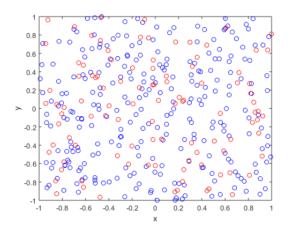R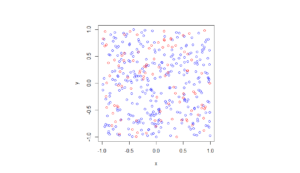Python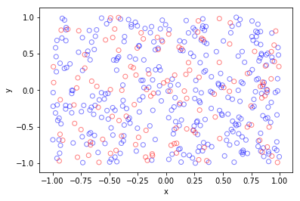##### Spatially dependent thinning

Observe how there are more thinned points (that is, red circles) near the origin, which is of course where the thinning function $$p(x)=\exp(-|x|^2/s^2)$$ attains its maximum.

MATLAB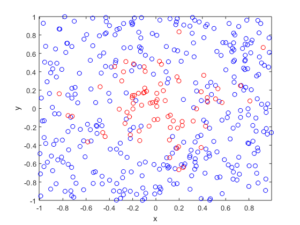R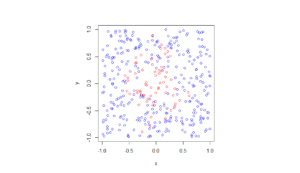Python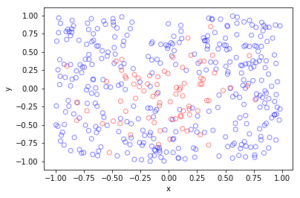The thinning operation is covered in Stochastic Geometry and its Applications by Chiu, Stoyan, Kendall and Mecke (Chapter 5). It is also covered in the book Statistical Inference and Simulation for Spatial Point Processes by Moller and Waagepetersen (Section 3.2.2). Kallenberg presents a more theoretical and rigorous take on thinning Poisson point processes in of his new book Random Measures, Theory and Applications (Chapter 3). (A point process can be interpreted as a type of random measure called a random counting measure because it gives the random number of points in a set.)

Thinning is also covered in books that apply point processes to wireless networks, such as Stochastic Geometry and Wireless Networks by Baccelli and Błaszczyszyn (Volume 1, Section 1.3.2) or Stochastic Geometry for Wireless Networks (Section 2.7.3) by Haenggi. These books also give examples of thinning point processes for wireless network applications.

## Simulating a Thomas cluster point process

Sometimes with just a little tweaking of a point process, you can get a new  point process. An example of this is the Thomas point process, which is a type of cluster point process, meaning that its randomly located points tend to form random clusters.  This point process is an example of a family of cluster point processes known as Neyman-Scott point processes, which have been used as models in spatial statistics and telecommunications. If that sounds familiar, that is because this point process is very similar to the Matérn point cluster process, which I covered in the previous post

The only difference between the two point processes is how the points are randomly located. In each cluster of a Thomas point process, each individual point is located according to two independent zero-mean normal variables with variance $$\sigma^2$$, describing the $$x$$ and $$y$$ coordinates relative to the cluster centre, whereas each point of a Matérn point process is located uniformly in a disk.

Working in polar coordinates, an equivalent way to simulate a Thomas point process is to use independent and identically-distributed Rayleigh random variables for the radial (or $$\rho$$) coordinates, instead of using random variables with a triangular distribution, which are used to simulate the Matérn point process. This method works because in polar coordinates a uniform random variable for the angular (or $$\theta$$ ) coordinate and a Rayleigh random variable for the angular (or $$\rho$$) is equivalent to in Cartesian coordinates two independent zero-mean normal variables. This is exactly the trick behind the Box-Muller transform for generating normal random variables using just uniform random variables.

If you’re familiar with simulating the Matérn point process, you can skip the next couple sections, and just read how the points are located.

## Overview

Simulating a Thomas cluster point process requires first simulating a homogeneous Poisson point process with intensity $$\lambda>0$$ on some simulation window, such as a rectangle, which is the simulation window I will use here. Then for each point of this underlying point process, simulate a Poisson number of points with mean $$\mu>0$$, and for each point simulate two independent zero-mean normal variables with variance $$\sigma^2$$, corresponding to the (relative) Cartesian coordinates .

The underlying  point process is sometimes called the parent (point) process, and its points are centres of the cluster disks. The subsequent point process on all the disks is called daughter (point) process and it forms the clusters. I have already written about simulating the homogeneous Poisson point processes on a rectangle and a disk, so  those posts are good starting points, and I will not focus  too much on details for these steps steps.

Importantly, like the Matérn point process, it’s possible for daughter points to appear in the simulation window that come from parents points outside the simulation window. To handle these edge effects, the point processes must be first simulated on an extended version of the simulation window. Then only the daughter points within the simulation window are kept and the rest are removed.

We can add a strip of some width $$d$$ all around the simulation window. But what value does $$d$$ take? Well, in theory, it is possible that a daughter point comes from a parent point that is very far from the simulation window. But that probability becomes vanishingly small as the distance increases, due to the daughter points being located according to zero-mean normal random variables.

For example, if a single parent is at a distance $$d=6 \sigma$$ from the simulation, then there is about a $1/1 000 000 000$ chance that a single daughter point will land in the simulation window. The probability is simply $$1-\Phi(6 \sigma)$$, where $$\Phi$$ is the cumulative distribution function of a normal variable with zero mean and standard deviation $$\sigma>0$$.  This is what they call a six sigma event.  In my code, I set $$d=6 \sigma$$, but $$d=4 \sigma$$ is good enough, which is the value that the R library spatstat uses by default.

Due to this approximation, this simulation cannot be called a perfect simulation, despite the approximation being highly accurate. In practice, it will not have no measurable effect on simulation results, as the number of simulations will rarely be high enough for (hypothetical) daughter points to come from (hypothetical) parent points outside the window.

## Steps

##### Number of points

Simulate the underlying or parent Poisson point process on the rectangle with $$N_P$$ points. Then for each point, simulate a Poisson number of points, where each disk now has $$D_i$$ number of points. Then the total number of points is simply $$N=D_1+\dots +D_{P}=\sum_{i=1}^{N_P}D_i$$. The random variables $$P$$ and $$D_i$$ are Poisson random variables with respective means $$\lambda A$$ and $$\mu$$, where $$A$$ is the area of the rectangular simulation window. To simulate these random variables in MATLAB, use the poissrnd function. To do this in R, use the  standard function rpois. In Python, we can use either functions scipy.stats.poisson or numpy.random.poisson from the SciPy or NumPy libraries.

##### Locations of points

The points of the parent point process are randomly positioned by using Cartesian coordinates. For a homogeneous Poisson point process, the $$x$$ and $$y$$ coordinates of each point are independent uniform points, which is also the case for the binomial point process, covered in an earlier post.

As mentioned in the introduction of this post, the points of all the daughter point process are randomly positioned by either using polar  coordinates or Cartesian coordinates, due to the Box-Muller transform. But because we ultimately convert back to Cartesian coordinates (for example, to plot the points), we will work entirely in this coordinate system.  Each point is then simply positioned with two independent zero-mean normal random variables, representing the $$x$$ and $$y$$ coordinates relative to the original parent point.

##### Shifting all the points in each cluster disk

In practice (that is, in the code), all the daughter points are simulated relative to the origin. Then for each cluster disk, all the points need to be shifted, so the origin coincides with the parent point, which completes the simulation step.

To use vectorization in the code,  the coordinates of each cluster point are repeated by the number of daughters in the corresponding cluster by using the functions repelem in MATLAB, rep in R, and repeat in Python.

## Code

I have implemented the simulation procedure in MATLAB, R and Python, which as usual are all very similar. The code can be downloaded here.

##### MATLAB
 %Simulation window parameters xMin=-.5; xMax=.5; yMin=-.5; yMax=.5; %Parameters for the parent and daughter point processes lambdaParent=10;%density of parent Poisson point process lambdaDautgher=100;%mean number of points in each cluster sigma=0.05;%sigma for normal variables (ie random locations) of daughters %Extended simulation windows parameters rExt=7*sigma; %extension parameter -- use factor of deviation %for rExt, use factor of deviation sigma eg 6 or 7 xMinExt=xMin-rExt; xMaxExt=xMax+rExt; yMinExt=yMin-rExt; yMaxExt=yMax+rExt; %rectangle dimensions xDeltaExt=xMaxExt-xMinExt; yDeltaExt=yMaxExt-yMinExt; areaTotalExt=xDeltaExt*yDeltaExt; %area of extended rectangle %Simulate Poisson point process for the parents numbPointsParent=poissrnd(areaTotalExt*lambdaParent,1,1);%Poisson number %x and y coordinates of Poisson points for the parent xxParent=xMinExt+xDeltaExt*rand(numbPointsParent,1); yyParent=yMinExt+yDeltaExt*rand(numbPointsParent,1); %Simulate Poisson point process for the daughters (ie final poiint process) numbPointsDaughter=poissrnd(lambdaDautgher,numbPointsParent,1); numbPoints=sum(numbPointsDaughter); %total number of points %Generate the (relative) locations in Cartesian coordinates by %simulating independent normal variables xx0=normrnd(0,sigma,numbPoints,1); yy0=normrnd(0,sigma,numbPoints,1); %replicate parent points (ie centres of disks/clusters) xx=repelem(xxParent,numbPointsDaughter); yy=repelem(yyParent,numbPointsDaughter); %translate points (ie parents points are the centres of cluster disks) xx=xx(:)+xx0; yy=yy(:)+yy0; %thin points if outside the simulation window booleInside=((xx&gt;=xMin)&amp;(xx&lt;=xMax)&amp;(yy&gt;=yMin)&amp;(yy&lt;=yMax)); %retain points inside simulation window xx=xx(booleInside); yy=yy(booleInside); %Plotting scatter(xx,yy);
##### R

Note: it is a bit tricky to write “<-” in the R code (as it automatically changes to the html equivalent in the HTML editor I am using), so I have usually used “=” instead of the usual “<-”.

 #Simulation window parameters xMin=-.5; xMax=.5; yMin=-.5; yMax=.5; #Parameters for the parent and daughter point processes lambdaParent=10;#density of parent Poisson point process lambdaDaughter=100;#mean number of points in each cluster sigma=0.05; #sigma for normal variables (ie random locations) of daughters #Extended simulation windows parameters rExt=7*sigma; #extension parameter #for rExt, use factor of deviation sigma eg 6 or 7 xMinExt=xMin-rExt; xMaxExt=xMax+rExt; yMinExt=yMin-rExt; yMaxExt=yMax+rExt; #rectangle dimensions xDeltaExt=xMaxExt-xMinExt; yDeltaExt=yMaxExt-yMinExt; areaTotalExt=xDeltaExt*yDeltaExt; #area of extended rectangle #Simulate Poisson point process for the parents numbPointsParent=rpois(1,areaTotalExt*lambdaParent);#Poisson number of points #x and y coordinates of Poisson points for the parent xxParent=xMinExt+xDeltaExt*runif(numbPointsParent); yyParent=yMinExt+yDeltaExt*runif(numbPointsParent); #Simulate Poisson point process for the daughters (ie final poiint process) numbPointsDaughter=rpois(numbPointsParent,lambdaDaughter); numbPoints=sum(numbPointsDaughter); #total number of points #Generate the (relative) locations in Cartesian coordinates by #simulating independent normal variables xx0=rnorm(numbPoints,0,sigma); yy0=rnorm(numbPoints,0,sigma); #replicate parent points (ie centres of disks/clusters) xx=rep(xxParent,numbPointsDaughter); yy=rep(yyParent,numbPointsDaughter); #translate points (ie parents points are the centres of cluster disks) xx=xx+xx0; yy=yy+yy0; #thin points if outside the simulation window booleInside=((xx&gt;=xMin)&amp;(xx&lt;=xMax)&amp;(yy&gt;=yMin)&amp;(yy&lt;=yMax)); #retain points inside simulation window xx=xx[booleInside]; yy=yy[booleInside]; #Plotting par(pty="s") plot(xx,yy,'p',xlab='x',ylab='y',col='blue');

Of course, as I have mentioned before, simulating a spatial point processes in R is even easier with the powerful spatial statistics library spatstat. The Thomas cluster point process is simulated by using the function rThomas, but other cluster point processes, including Neyman-Scott types, are possible.

##### Python

Note: in previous posts I used the SciPy functions for random number generation, but now use the NumPy ones, but there is little difference, as SciPy builds off NumPy.

 import numpy as np;&nbsp; # NumPy package for arrays, random number generation, etc import matplotlib.pyplot as plt&nbsp; # For plotting # Simulation window parameters xMin = -.5; xMax = .5; yMin = -.5; yMax = .5; # Parameters for the parent and daughter point processes lambdaParent = 10; # density of parent Poisson point process lambdaDaughter = 100; # mean number of points in each cluster sigma = 0.05; # sigma for normal variables (ie random locations) of daughters #Extended simulation windows parameters rExt=7*sigma; #extension parameter #for rExt, use factor of deviation sigma eg 6 or 7 xMinExt = xMin - rExt; xMaxExt = xMax + rExt; yMinExt = yMin - rExt; yMaxExt = yMax + rExt; # rectangle dimensions xDeltaExt = xMaxExt - xMinExt; yDeltaExt = yMaxExt - yMinExt; areaTotalExt = xDeltaExt * yDeltaExt; # area of extended rectangle # Simulate Poisson point process for the parents numbPointsParent = np.random.poisson(areaTotalExt * lambdaParent);# Poisson number of points # x and y coordinates of Poisson points for the parent xxParent = xMinExt + xDeltaExt * np.random.uniform(0, 1, numbPointsParent); yyParent = yMinExt + yDeltaExt * np.random.uniform(0, 1, numbPointsParent); # Simulate Poisson point process for the daughters (ie final poiint process) numbPointsDaughter = np.random.poisson(lambdaDaughter, numbPointsParent); numbPoints = sum(numbPointsDaughter); # total number of points # Generate the (relative) locations in Cartesian coordinates by # simulating independent normal variables xx0 = np.random.normal(0, sigma, numbPoints); # (relative) x coordinaets yy0 = np.random.normal(0, sigma, numbPoints); # (relative) y coordinates # replicate parent points (ie centres of disks/clusters) xx = np.repeat(xxParent, numbPointsDaughter); yy = np.repeat(yyParent, numbPointsDaughter); # translate points (ie parents points are the centres of cluster disks) xx = xx + xx0; yy = yy + yy0; # thin points if outside the simulation window booleInside=((xx&gt;=xMin)&amp;(xx&lt;=xMax)&amp;(yy&gt;=yMin)&amp;(yy&lt;=yMax)); # retain points inside simulation window xx = xx[booleInside]; yy = yy[booleInside]; # Plotting plt.scatter(xx, yy, edgecolor='b', facecolor='none', alpha=0.5); plt.xlabel("x"); plt.ylabel("y"); plt.axis('equal');
##### Julia

After writing this post, I later wrote the code in Julia. The code is here and my thoughts about Julia are here.

## Results

The results show that the clusters of Thomas point process tend to be more blurred than those of Matérn point process, which has cluster edges clearly defined by the disks.  The points of of a Thomas point process can be far away from the centre of each cluster, depending on the variance of the normal random variables used in the simulation.

##### MATLAB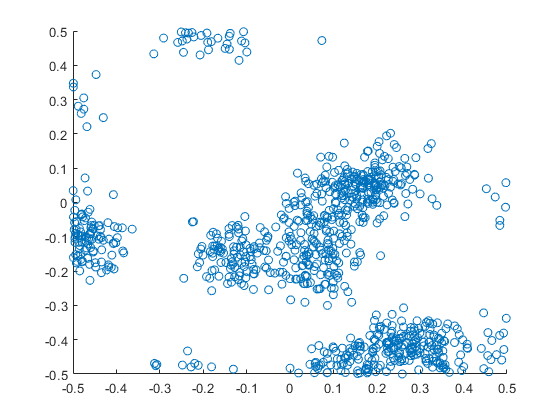##### R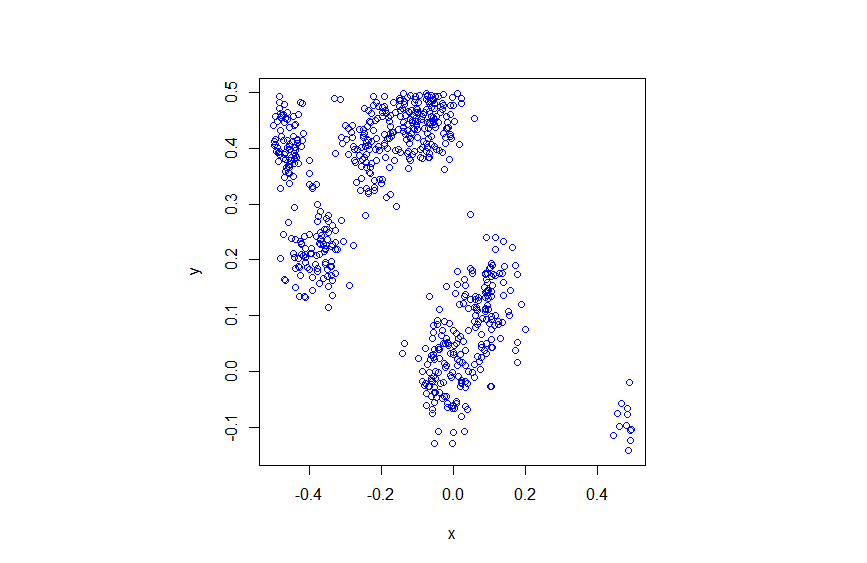##### Python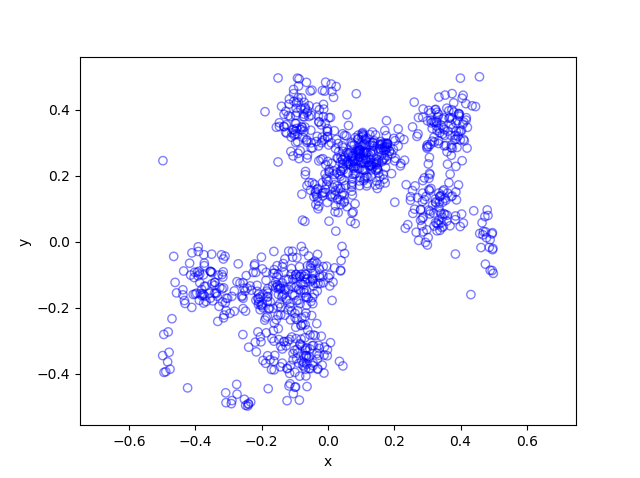Thomas (and Matérn) cluster point processes and more generally Neyman-Scott point processes are covered in standard books on the related fields of spatial statistics, point processes and stochastic geometry, such as the following: Spatial Point Patterns: Methodology and Applications with R by  Baddeley, Rubak and Turner, on page 461; Statistical Analysis and Modelling of Spatial Point Patterns Statistics by Illian, Penttinen, Stoyan, amd Stoyan, page 370 and Section 6.3.2; Stochastic Geometry and its Applications by Chiu, Stoyan, Kendall and Mecke,  on page 173; and; Statistical Inference and Simulation for Spatial Point Processes by Møller and Waagepetersen, in Section 5.3. I would probably recommend the first two books for beginners.

The Thomas point process has also appeared in models of wireless networks, which we covered in the book Stochastic Geometry Analysis of Cellular Networks by Błaszczyszyn, Haenggi, Keeler, and Mukherjee, Section 8.1.8.

More generally, Neyman-Scott point processes belong to a family of point processes called shot noise Cox point processes; see the paper by Møller.

There is the book Spatial Point Patterns: Methodology and Applications with R written by spatial statistics experts Baddeley, Rubak and Turner, which covers the spatial statistics (and point process simulation) R-package spatstat.

In my travels on the web, I found this post where the writer also simulates the Thomas and Matérn point processes in Python, independent of my code.  That code is a bit different to mine because I use the repeat function and simulate all the Poisson variables at once, instead of using a for-loop and simulating a Poisson variable for each iteration.  I also think it’s not quite correct because I do not see how they account for edge effects.

## Simulating a Matérn cluster point process

A Matérn cluster point process is a type of cluster point process, meaning that its randomly located points tend to form random clusters. (I skip the details here, but by using techniques from spatial statistics, it is possible to make the definition of clustering more precise.) This point process is an example of a family of cluster point processes known as Neyman-Scott point processes, which have been used in spatial statistics and telecommunications.

I should point out that the Matérn cluster point process should not be confused with the Matérn hard-core point process, which is a completely different type of point process. (For a research article, I have actually written code in MATLAB that simulates this type of point process.) Bertril Matérn proposed at least four types of point processes, and his name also refers to a specific type of covariance function used to define Gaussian processes.

## Overview

Simulating a Matérn cluster point process requires first simulating  a homogeneous Poisson point process with an intensity $$\lambda>0$$ on some simulation window, such as a rectangle, which is the simulation window I will use here. Then for each point of this underlying point process, simulate a Poisson number of points with mean $$\mu>0$$ uniformly on a disk with a constant radius $$r>0$$. The underlying  point process is sometimes called the parent (point) process, and its points are centres of the cluster disks.

The subsequent point process on all the disks is called daughter (point) process and it forms the clusters. I have already written about simulating the homogeneous Poisson point processes on a rectangle and a disk, so those posts are good starting points, and I will not focus  too much on details for these steps.

### Edge effects

The main challenge behind sampling this point process, which I originally forgot about in an earlier version of this post, is that it’s possible for daughter points to appear in the simulation window that come from parents points outside the simulation window. In other words, parents points outside the simulation window contribute to points inside the window.

To remove these edge effects, the point processes must be simulated on an extended version of the simulation window. Then only the daughter points within the simulation window are kept and the rest are removed.  Consequently, the points are simulated on an extended window, but we only see the points inside the simulation window.

To create the extended simulation window, we can add a strip of width $$r$$ all around the simulation window. Why? Well, the distance $$r$$ is the maximum distance from the simulation window that a possibly contributing parent point (outside the simulation window) can exist, while still having daughter points inside the simulation window. This means it is impossible for a hypothetical parent point beyond this distance (outside the extended window) to generate a daughter point that can fall inside the simulation window.

## Steps

##### Number of points

Simulate the underlying or parent Poisson point process on the rectangle with $$N_P$$ points. Then for each point, simulate a Poisson number of points, where each disk now has $$D_i$$ number of points. Then the total number of points is simply $$N=D_1+\dots +D_{P}=\sum_{i=1}^{N_P}D_i$$. The random variables $$P$$ and $$D_i$$ are Poisson random variables with respective means $$\lambda A$$ and $$\mu$$, where $$A$$ is the area of the rectangular simulation window. To simulate these random variables in MATLAB, use the poissrnd function. To do this in R, use the  standard function rpois. In Python, we can use either functions scipy.stats.poisson or numpy.random.poisson from the SciPy or NumPy libraries.

##### Locations of points

The points of the parent point process are randomly positioned by using Cartesian coordinates. For a homogeneous Poisson point process, the $$x$$ and $$y$$ coordinates of each point are independent uniform points, which is also the case for the binomial point process, covered in a previous post. The points of all the daughter point process are randomly positioned by using polar  coordinates. For a homogeneous Poisson point process, the $$\theta$$ and $$\rho$$ coordinates of each point are independent  variables,  respectively with uniform and triangle distributions, which was covered in a previous post. Then we  convert coordinates back to Cartesian form, which is easily done in MATLAB with the pol2cart function. In languages without such a function: $$x=\rho\cos(\theta)$$ and $$y=\rho\sin(\theta)$$.

##### Shifting all the points in each cluster disk

In practice (that is, in the code), all the daughter points are simulated in a disk with its centre at the origin. Then for each cluster disk, all the points have to be shifted to the origin is the center of the cluster, which completes the simulation step.

To use vectorization in the code,  the coordinates of each cluster point are repeated by the number of daughters in the corresponding cluster by using the functions repelem in MATLAB, rep in R, and repeat in Python.

## Code

I’ll now give some code in MATLAB, R and Python, which you can see are all very similar. It’s also located here.

##### MATLAB

The MATLAB code is located here.

#### R

The R code is located here.

Of course, as I have mentioned before, simulating a spatial point processes in R is even easier with the powerful spatial statistics library spatstat. The Matérn cluster point process is simulated by using the function rMatClust, but other cluster point processes, including Neyman-Scott types, are possible.

##### Python

The Pyyhon code is located here.

Note: in previous posts I used the SciPy functions for random number generation, but now use the NumPy ones, but there is little difference, as SciPy builds off NumPy.

##### Julia

After writing this post, I later wrote the code in Julia. The code is here and my thoughts about Julia are here.

## Results

##### MATLAB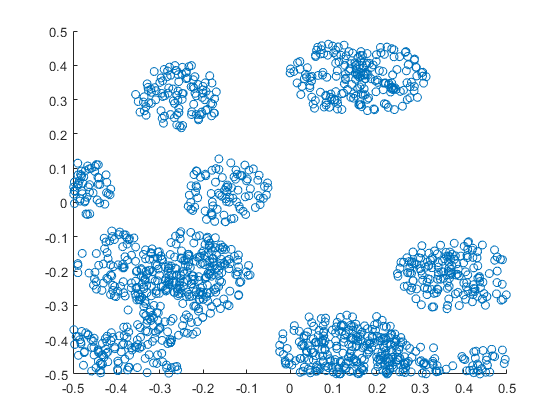##### R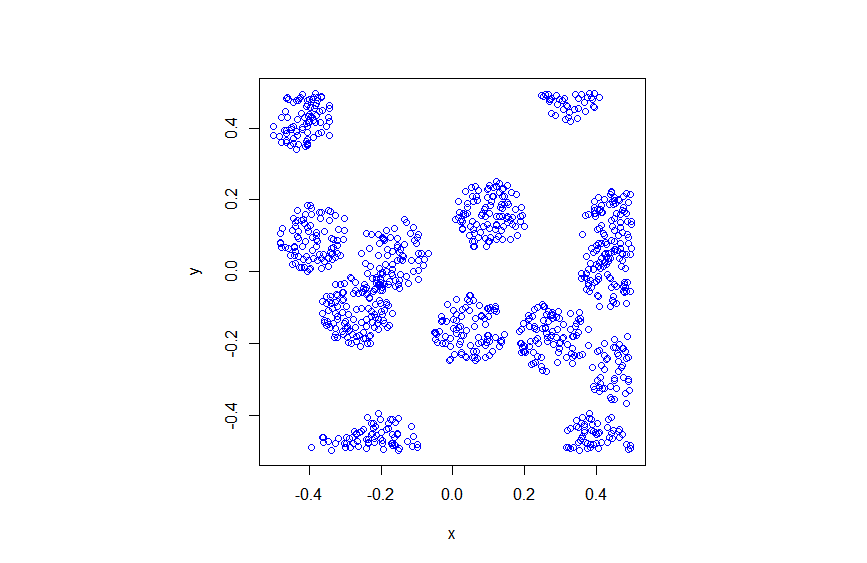##### Python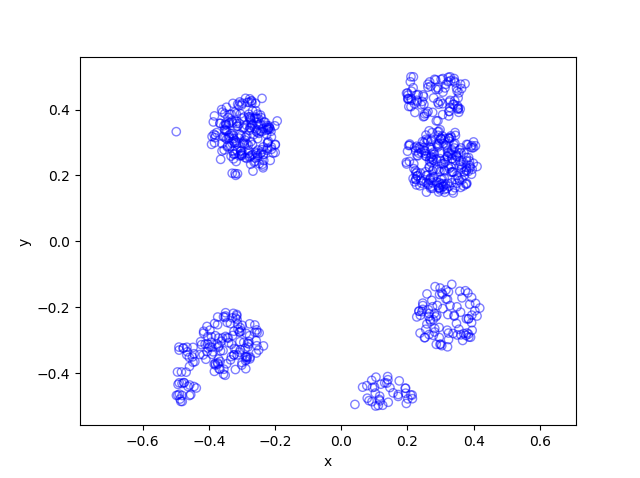Matérn cluster point processes and more generally Neyman-Scott point processes are covered in standard books on the related fields of spatial statistics, point processes and stochastic geometry, such as the following: Spatial Point Patterns: Methodology and Applications with R by  Baddeley, Rubak and Turner, on page 461; Statistical Analysis and Modelling of Spatial Point Patterns Statistics by Illian, Penttinen, Stoyan, amd Stoyan, Section 6.3.2, starting on page 370; Stochastic Geometry and its Applications by Chiu, Stoyan, Kendall and Mecke,  on page 173; and; Statistical Inference and Simulation for Spatial Point Processes by Moller and Waagepetersen, in Section 5.3. I would probably recommend the first two books for beginners.

The Matérn point process has also appeared in models of wireless networks, which we covered in the book Stochastic Geometry Analysis of Cellular Networks by Błaszczyszyn, Haenggi, Keeler, and Mukherjee, Section 8.1.8.

There is the book Spatial Point Patterns: Methodology and Applications with R written by spatial statistics experts  Baddeley, Rubak and Turner, which covers the spatial statistics (and point process simulation) R-package spatstat.

## Simulating a Poisson point process on a triangle

The title gives it away. But yes, after  two posts about simulating a Poisson point process  on a rectangle and disk, the next shape is a triangle. Useful, right?

Well, I actually had to do this once for a part of something larger. You can divide  polygons, regular or irregular, into triangles, which is often called triangulation, and there is much code that does triangulation.  Using the independence property of the Poisson process, you can then simulate a Poisson point process on each triangle, and you end up with a Poisson point process on a polygon.

But the first step is to do it on a triangle. Consider a general triangle with its three corners labelled $$\textbf{A}$$, $$\textbf{B}$$ and $$\textbf{C}$$. Again, simulating a Poisson point process comes down to the number of points and the locations of points.

### Method

##### Number of points

The number of points of a homogeneous Poisson point process  in any shape with with area $$A$$ is simply a Poisson random variable with mean  $$\lambda A$$, where $$A$$ is the area of the shape. For the triangle’s area, we just uses Herron’s formula, which says that a triangle with sides $$a$$, $$b$$, and $$c$$  has the area $$A=\sqrt{s(s-a)(s-b)(s-c)}$$, where $$s=(a+b+c)/2$$, which means you just need to use Pythagoras’ theorem for  the lengths $$a$$, $$b$$, and $$c$$. Interestingly, this standard formula can be prone to numerical error if the triangle is very thin or needle-shaped. A more secure and stable expression is

$$A=\frac{1}{4}\sqrt{ (a+(b+c)) (c-(a-b)) (c+(a-b)) (a+(b-c)) },$$

where the brackets do matter; see Section 2.5 in the book by Higham.

Of course in MATLAB you can just use the function polyarea or the function with the same name in R.

Now just generate or simulate Poisson random variables with mean (or parameter)  $$\lambda A$$. In MATLAB,  use the poissrnd function with the argument $$\lambda A$$. In R, it is done similarly with the standard  function rpois . In Python, we can use either the scipy.stats.poisson or numpy.random.poisson function from the SciPy or NumPy libraries.

##### Locations of points

We need to position all the points randomly and uniformly on a triangle.  As with the previous two simulation cases, to position a single point $$(x, y)$$, you first need to produce two random uniform variables on the unit interval $$(0,1)$$, say $$U$$ and $$V$$. I’ll denote the $$x$$ and $$y$$ coordinates of point by $$x_{\textbf{A}}$$ and $$y_{\textbf{A}}$$, and similarly for the other two points.  To get the random $$x$$ and $$y$$ values, you use these two formulas:

$$x=\sqrt{U} x_{\textbf{A}}+\sqrt{U}(1-V x_{\textbf{B}})+\sqrt{U}V x_{\textbf{C}}$$

$$y=\sqrt{U} y_{\textbf{A}}+\sqrt{U}(1-V y_{\textbf{B}})+\sqrt{U}V y_{\textbf{C}}$$

Done. A Poisson point process simulated on a triangle .

### Code

I now present some code in MATLAB, R and Python, which you can see are all very similar.  To avoid using a for-loop and employing instead MATLAB’s inbuilt vectorization, I use the dot notation for the product $$\sqrt{U}V$$. In R and Python (using SciPy), that’s done automatically.

MATLAB


%Simulation window parameters -- points A,B,C of a triangle
xA=0;xB=1;xC=1; %x values of three points
yA=0;yB=0;yC=1; %y values of three points

%Point process parameters
lambda=100; %intensity (ie mean density) of the Poisson process

%calculate sides of trinagle
a=sqrt((xA-xB)^2+(yA-yB)^2);
b=sqrt((xB-xC)^2+(yB-yC)^2);
c=sqrt((xC-xA)^2+(yC-yA)^2);
s=(a+b+c)/2; %calculate semi-perimeter

%Use Herron's forumula -- or use polyarea
areaTotal=sqrt(s*(s-a)*(s-b)*(s-c)); %area of triangle

%Simulate Poisson point process
numbPoints=poissrnd(areaTotal*lambda);%Poisson number of points
U=(rand(numbPoints,1));%uniform random variables
V=(rand(numbPoints,1));%uniform random variables

xx=sqrt(U)*xA+sqrt(U).*(1-V)*xB+sqrt(U).*V*xC;%x coordinates of points
yy=sqrt(U)*yA+sqrt(U).*(1-V)*yB+sqrt(U).*V*yC;%y coordinates of points

%Plotting
scatter(xx,yy);
xlabel('x');ylabel('y');


R

Note: it is a bit tricky to write “<-” in the R code (as it automatically changes to the html equivalent in the HTML editor I am using), so I have usually used “=” instead of the usual “<-”.


#Simulation window parameters -- points A,B,C of a triangle
xA=0;xB=1;xC=1; #x values of three points
yA=0;yB=0;yC=1; #y values of three points

#Point process parameters
lambda=100; #intensity (ie mean density) of the Poisson process

#calculate sides of trinagle
a=sqrt((xA-xB)^2+(yA-yB)^2);
b=sqrt((xB-xC)^2+(yB-yC)^2);
c=sqrt((xC-xA)^2+(yC-yA)^2);
s=(a+b+c)/2; #calculate semi-perimeter

#Use Herron's forumula to calculate area
areaTotal=sqrt(s*(s-a)*(s-b)*(s-c)); #area of triangle

#Simulate a Poisson point process
numbPoints=rpois(1,areaTotal*lambda);#Poisson number of points
U=runif(numbPoints);#uniform random variables
V=runif(numbPoints);#uniform random variables

xx=sqrt(U)*xA+sqrt(U)*(1-V)*xB+sqrt(U)*V*xC;#x coordinates of points
yy=sqrt(U)*yA+sqrt(U)*(1-V)*yB+sqrt(U)*V*yC;#y coordinates of points

#Plotting
plot(xx,yy,'p',xlab='x',ylab='y',col='blue');


Simulating a Poisson point process in R is even easier, with the amazing spatial statistics library spatstat. You just need to define the triangular window.

#Simulation window parameters -- points A,B,C of a triangle
xA=0;xB=1;xC=1; #x values of three points
yA=0;yB=0;yC=1; #y values of three points

#Point process parameters
lambda=100; #intensity (ie mean density) of the Poisson process

library("spatstat");
#create triangle window object
windowTriangle=owin(poly=list(x=c(xA,xB,xC), y=c(yA,yB,yC)));
#create Poisson "point pattern" object
ppPoisson=rpoispp(lambda,win=windowTriangle)
plot(ppPoisson); #Plot point pattern object
#retrieve x/y values from point pattern object
xx=ppPoisson$x; yy=ppPoisson$y;


Python

Note: “lambda” is a reserved word in Python (and other languages), so I have used “lambda0” instead.

#import libraries
import numpy as np
import scipy.stats
import matplotlib.pyplot as plt

#Simulation window parameters -- points A,B,C of a triangle
xA=0;xB=1;xC=1; #x values of three points
yA=0;yB=0;yC=1; #y values of three points

#Point process parameters
lambda0=100; #intensity (ie mean density) of the Poisson process

#calculate sides of trinagle
a=np.sqrt((xA-xB)**2+(yA-yB)**2);
b=np.sqrt((xB-xC)**2+(yB-yC)**2);
c=np.sqrt((xC-xA)**2+(yC-yA)**2);
s=(a+b+c)/2; #calculate semi-perimeter

#Use Herron's forumula to calculate area -- or use polyarea
areaTotal=np.sqrt(s*(s-a)*(s-b)*(s-c)); #area of triangle

#Simulate a Poisson point process
numbPoints = scipy.stats.poisson(lambda0*areaTotal).rvs();#Poisson number of points
U = scipy.stats.uniform.rvs(0,1,((numbPoints,1)));#uniform random variables
V= scipy.stats.uniform.rvs(0,1,((numbPoints,1)));#uniform random variables

xx=np.sqrt(U)*xA+np.sqrt(U)*(1-V)*xB+np.sqrt(U)*V*xC;#x coordinates of points
yy=np.sqrt(U)*yA+np.sqrt(U)*(1-V)*yB+np.sqrt(U)*V*yC;#y coordinates of points

#Plotting
plt.scatter(xx,yy, edgecolor='b', facecolor='none', alpha=0.5 );
plt.xlabel("x"); plt.ylabel("y");


### Results

MATLAB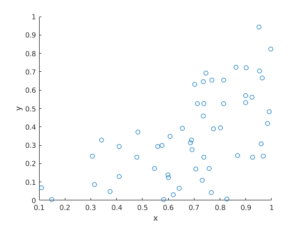R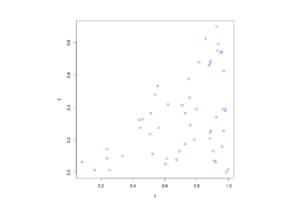Python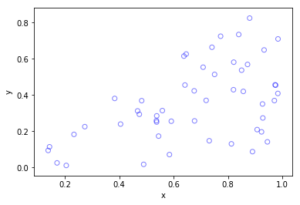The topic of placing a single point uniformly on a general triangle is discussed in this StackExchange post.  For the formulas, it cites the paper “Shape distributions” by Osada, Funkhouser, Chazelle and Dobkin”, where no proof is given.

I originally looked at placing single points in cells of a Dirichlet or Voronoi tesselation — terms vary. There is a lot of literature on this topic, particularly when the seeds of the cells form a Poisson point process. The references in the articles on Wikipedia and MathWorld are good starting points.

### Correction

In a previous version of this blog, there was an error in the two Cartesian formula for randomly simulating a point in a triangle. This has been fixed, but the error never existed in the code.

## Simulating a Poisson point process on a disk

Sometimes one needs to simulate a Poisson point process on a disk. I know I often do. A disk or disc, depending on your spelling preference, is isotropic or rotationally-invariant, so a lot of my simulations of a Poisson point process happen in a circular simulation window when I am considering such a setting. For example, maybe you want to consider a single wireless receiver in a Poisson network of wireless transmitters, which only cares about the distance to a transmitter. Alternatively, maybe you want to randomly sprinkle a virtual cake. What to do? A Poisson point process on a disk is the answer.

I will simulate a Poisson point process with intensity $$\lambda>0$$ on a disk with radius $$r>0$$. The simulation steps are very similar to those in the previous post where I simulated a  homogeneous Poisson point process on a rectangle, and I suggest going back to that post if you are not familiar with the material. The main difference between simulation on a rectangle and a disk is the positioning of the points, but first we’ll look at the number of points.

## Steps

##### Number of points

The number of points of a Poisson point process falling within a circle of radius $$r>0$$ is a Poisson random variable with mean  $$\lambda A$$, where $$A=\pi r^2$$ is the area of the disk. As in the rectangular case, this is the most complicated part of the simulation procedure. But as long as your preferred programming language can produce (pseudo-)random numbers according to a Poisson distribution, you can simulate a homogeneous Poisson point process on a disk.

To do this in MATLAB,  use the poissrnd function with the argument $$\lambda A$$. In R, it is done similarly with the standard  function rpois . In Python, we can use either the scipy.stats.poisson or numpy.random.poisson function from the SciPy or NumPy libraries.

### Locations of points

We need to position all the points randomly and uniformly on a disk. In the case of the rectangle, we worked in Cartesian coordinates. It is then natural that we now work in polar coordinates.  I’ll denote the angular and radial coordinate respectively by $$\theta$$ and $$\rho$$. To generate the random angular (or $$\theta$$) values, we simply produce uniform random variables between zero and one, which is what all standard (pseudo-)random number generators do in programming languages. But we then multiply all these numbers by $$2\pi$$, meaning that all the numbers now fall between $$0$$ and $$2\pi$$.

To generate the random radial (or $$\rho$$) values, a reasonable guess would be to do the same as before and generate uniform random variables between zero and one, and then multiply them by the disk radius $$r$$. But that would be wrong.

Before multiplying uniform random variables by the radius, we must take the square root of all the random numbers. We then multiply them by the radius, generating random variables between $$0$$ and $$r$$. (We must take the square root because the area element of a sector or disk is proportional to the radius squared, and not the radius.) These random numbers do not have a uniform distribution, due to the square root, but in fact their distribution is an example of the triangular distribution, which is defined with three real-valued parameters $$a$$, $$b$$ and $$c$$, and for our case, set $$a=0$$ and $$b=c=r$$.

In summary, if $$U$$ and $$V$$ are two independent uniform random variables on $$(0,1)$$, then random point located uniformly on a disk of radius $$r$$ has the polar coordinates $$(r\sqrt(U), 2\pi V)$$.

From polar to Cartesian coordinates

That’s it. We have generated polar coordinates for points randomly and uniformly located in a disk. But to plot the points, often we have to convert coordinates back to Cartesian form. This is easily done in MATLAB with the pol2cart function. In languages without such a function, trigonometry comes to the rescue: $$x=\rho\cos(\theta)$$ and $$y=\rho\sin(\theta)$$.

Equal x and y axes

Sometimes the plotted points more resemble points on an ellipse than a disk due to the different scaling of the x and y axes. To fix this in MATLAB, run the command: axis square. In Python, set axis(‘equal’) in your plot; see this page for a demonstration.

## Code

I’ll now give some code in MATLAB, R and Python, which you can see are all very similar

##### MATLAB
%Simulation window parameters
xx0=0; yy0=0; %centre of disk

areaTotal=pi*r^2; %area of disk

%Point process parameters
lambda=100; %intensity (ie mean density) of the Poisson process

%Simulate Poisson point process
numbPoints=poissrnd(areaTotal*lambda);%Poisson number of points
theta=2*pi*(rand(numbPoints,1)); %angular coordinates

%Convert from polar to Cartesian coordinates
[xx,yy]=pol2cart(theta,rho); %x/y coordinates of Poisson points

%Shift centre of disk to (xx0,yy0)
xx=xx+xx0;
yy=yy+yy0;

%Plotting
scatter(xx,yy);
xlabel('x');ylabel('y');
axis square;

##### R

Note: it is a bit tricky to write “<-” in the R code (as it automatically changes to the html equivalent in the HTML editor I am using), so I have usually used “=” instead of the usual “<-”.

#Simulation window parameters
xx0=0; yy0=0; #centre of disk

areaTotal=pi*r^2; #area of disk

#Point process parameters
lambda=100; #intensity (ie mean density) of the Poisson process

#Simulate Poisson point process
numbPoints=rpois(1,areaTotal*lambda);#Poisson number of points
theta=2*pi*runif(numbPoints);#angular  of Poisson points

#Convert from polar to Cartesian coordinates
xx=rho*cos(theta);
yy=rho*sin(theta);

#Shift centre of disk to (xx0,yy0)
xx=xx+xx0;
yy=yy+yy0;

#Plotting
par(pty="s")
plot(xx,yy,'p',xlab='x',ylab='y',col='blue');


Of course, with the amazing spatial statistics library spatstat, simulating a Poisson point process in R is even easier.

library("spatstat"); #load spatial statistics library
#create Poisson "point pattern" object
plot(ppPoisson); #Plot point pattern object
#retrieve x/y values from point pattern object
xx=ppPoisson$x; yy=ppPoisson$y;


Actually, you can even do it all in two lines: one for loading the spatstat library and one for creating and plotting the point pattern object.

##### Python

Note: “lambda” is a reserved word in Python (and other languages), so I have used “lambda0” instead.

import numpy as np; #NumPy package for arrays, random number generation, etc
import matplotlib.pyplot as plt #for plotting

#Simulation window parameters
xx0=0; yy0=0; #centre of disk
areaTotal=np.pi*r**2; #area of disk

#Point process parameters
lambda0=100; #intensity (ie mean density) of the Poisson process

#Simulate Poisson point process
numbPoints = np.random.poisson(lambda0*areaTotal);#Poisson number of points
theta=2*np.pi*np.random.uniform(0,1,numbPoints); #angular coordinates

#Convert from polar to Cartesian coordinates
xx = rho * np.cos(theta);
yy = rho * np.sin(theta);

#Shift centre of disk to (xx0,yy0)
xx=xx+xx0; yy=yy+yy0;

#Plotting
plt.scatter(xx,yy, edgecolor='b', facecolor='none', alpha=0.5 );
plt.xlabel("x"); plt.ylabel("y");
plt.axis('equal');

##### Julia

After writing this post, I later wrote the code in Julia. The code is here and my thoughts about Julia are here.

## Results

##### Python

The third edition of the classic book Stochastic Geometry and its Applications by Chiu, Stoyan, Kendall and Mecke details on page 54 how to uniformly place points on a disk, which they call the radial way. The same simulation section appears in the previous edition by Chiu, Kendall and Mecke (Chiu didn’t appear as an author until the current edition), though these books in general have little material on simulation methods. There is the book Spatial Point Patterns: Methodology and Applications with R written by spatial statistics experts  Baddeley, Rubak and Turner, which covers the spatial statistics (and point process simulation) R-package spatstat.

## Simulating a homogeneous Poisson point process on a rectangle

This is the first of a series of posts about simulating Poisson point processes. I’ll start with arguably the simplest Poisson point process on two-dimensional space, which is the homogeneous one defined on a rectangle. Let’s say that we we want to simulate a Poisson point process with intensity $$\lambda>0$$ on a (bounded) rectangular region, for example, the rectangle $$[0,w]\times[0,h]$$ with dimensions $$w>0$$ and $$h>0$$ and area $$A=wh$$. We assume for now that the bottom left corner of the rectangle is at the origin.

## Steps

##### Number of points

The number of points in the rectangle  $$[0,w]\times[0,h]$$ is a Poisson random variable with mean $$\lambda A$$. In other words, this random variable is distributed according to the Poisson distribution with parameter $$\lambda A$$, and not just $$\lambda$$, because the number of points depends on the size of the simulation region.

This is the most complicated part of the simulation procedure. As long as your preferred programming language can produce (pseudo-)random numbers according to a Poisson distribution, you can simulate a homogeneous Poisson point process. There’s a couple of different ways used to simulate Poisson random variables, but we will skip the details. In MATLAB, it is done by using the poissrnd function with the argument $$\lambda A$$. In R, it is done similarly with the standard  function rpois . In Python, we can use either the scipy.stats.poisson or numpy.random.poisson function from the SciPy or NumPy libraries.

##### Location of points

The points now need to be positioned randomly, which is done by using Cartesian coordinates. For a homogeneous Poisson point process, the $$x$$ and $$y$$ coordinates of each point are independent uniform points, which is also the case for the binomial point process, covered in a previous post. For the rectangle $$[0,w]\times[0,h]$$, the $$x$$ coordinates are uniformly sampled on the interval $$[0,w]$$, and similarly for the $$y$$ coordinates. If the bottom left corner of rectangle is located at the point $$(x_0,y_0)$$, then we just have to shift the random $$x$$ and $$y$$ coordinates by respectively adding $$x_0$$ and $$y_0$$.

Every scientific programming language has a random uniform number generator because it is the default random number generator. In MATLAB, R and SciPy, it is respectively rand, runif and scipy.stats.uniform.

## Code

Here is some code that I wrote for simulating a homogeneous Poisson point process on a rectangle. You will notice that in all the code samples the part that simulates the Poisson point process requires only three lines of code: one line for the number of points and two lines lines for the $$x$$ and $$y$$ coordinates  of the points.

##### MATLAB
%Simulation window parameters
xMin=0;xMax=1;
yMin=0;yMax=1;
xDelta=xMax-xMin;yDelta=yMax-yMin; %rectangle dimensions
areaTotal=xDelta*yDelta;

%Point process parameters
lambda=100; %intensity (ie mean density) of the Poisson process

%Simulate Poisson point process
numbPoints=poissrnd(areaTotal*lambda);%Poisson number of points
xx=xDelta*(rand(numbPoints,1))+xMin;%x coordinates of Poisson points
yy=xDelta*(rand(numbPoints,1))+yMin;%y coordinates of Poisson points

%Plotting
scatter(xx,yy);
xlabel('x');ylabel('y');

##### R

Note: it is a bit tricky to write “<-” in the R code (as it automatically changes to the html equivalent in the HTML editor I am using), so I have usually used “=” instead of the usual “<-”.

#Simulation window parameters
xMin=0;xMax=1;
yMin=0;yMax=1;
xDelta=xMax-xMin;yDelta=yMax-yMin; #rectangle dimensions
areaTotal=xDelta*yDelta;

#Point process parameters
lambda=100; #intensity (ie mean density) of the Poisson process

#Simulate Poisson point process
numbPoints=rpois(1,areaTotal*lambda);#Poisson number of points
xx=xDelta*runif(numbPoints)+xMin;#x coordinates of Poisson points
yy=xDelta*runif(numbPoints)+yMin;#y coordinates of Poisson points

#Plotting
plot(xx,yy,'p',xlab='x',ylab='y',col='blue');

##### Python

Note: “lambda” is a reserved word in Python (and other languages), so I have used “lambda0” instead.

import numpy as np
import scipy.stats
import matplotlib.pyplot as plt

#Simulation window parameters
xMin=0;xMax=1;
yMin=0;yMax=1;
xDelta=xMax-xMin;yDelta=yMax-yMin; #rectangle dimensions
areaTotal=xDelta*yDelta;

#Point process parameters
lambda0=100; #intensity (ie mean density) of the Poisson process

#Simulate Poisson point process
numbPoints = scipy.stats.poisson( lambda0*areaTotal ).rvs()#Poisson number of points
xx = xDelta*scipy.stats.uniform.rvs(0,1,((numbPoints,1)))+xMin#x coordinates of Poisson points
yy = yDelta*scipy.stats.uniform.rvs(0,1,((numbPoints,1)))+yMin#y coordinates of Poisson points
#Plotting
plt.scatter(xx,yy, edgecolor='b', facecolor='none', alpha=0.5 )
plt.xlabel("x"); plt.ylabel("y")

##### Julia

After writing this post, I later wrote the code in Julia. The code is here and my thoughts about Julia are here.

## Results

##### MATLAB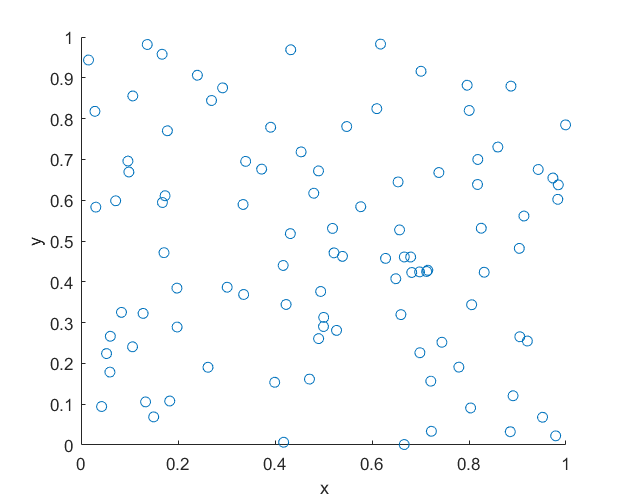##### R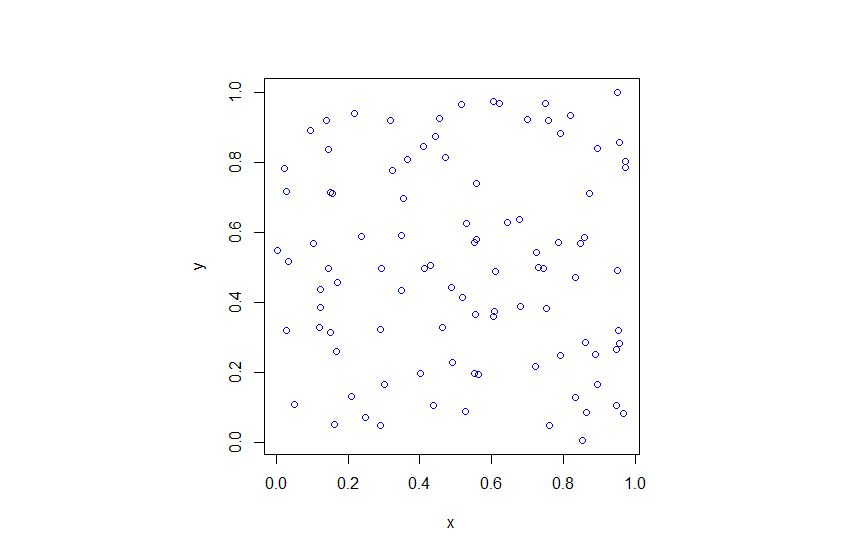##### Python